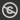Science, Maths & Technology
Free course

# Introducing vectors for engineering applications

Free statement of participation on completion## Course reviews

Applied mathematics is a key skill for practicing engineers and mathematical modelling is an ever-increasing field within engineering. This free course, Introducing vectors for engineering applications, covers one aspect of a Level 1 engineering module, the application of vectors and vector algebra, using examples inspired by engineering applications.

## Course learning outcomes

After studying this course, you should be able to:

• identify if a quantity is a vector
• represent vectors from engineering problems in an appropriate form
• model simple engineering systems (such as combining forces) using vectors
• perform simple algebraic procedures using vectors.

First Published: 04/11/2021

Updated: 04/11/2021

Skip Rate and Review